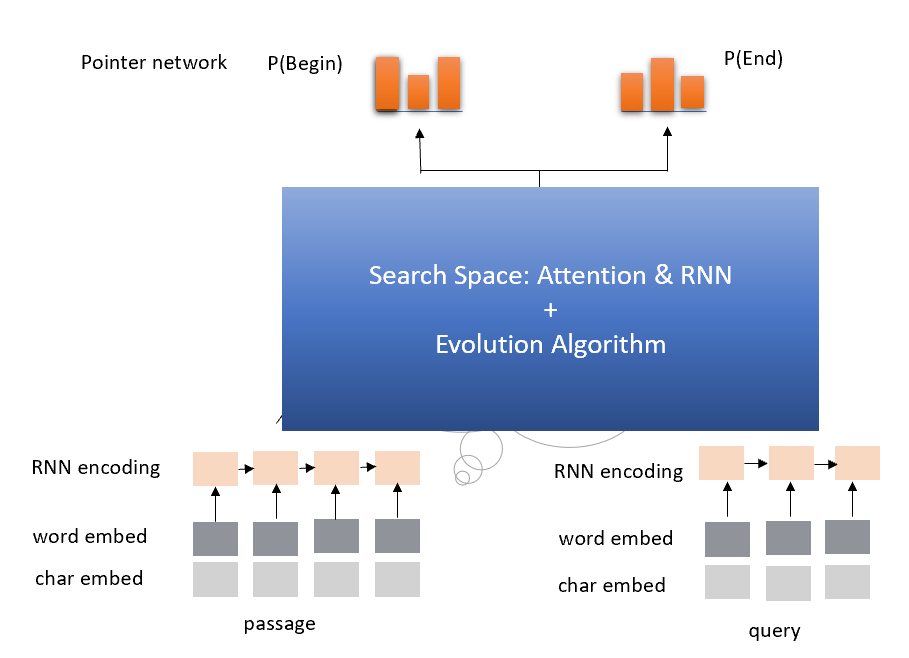# Automatic Model Architecture Search for Reading Comprehension¶

This example shows us how to use Genetic Algorithm to find good model architectures for Reading Comprehension.

## 1. Search Space¶

Since attention and RNN have been proven effective in Reading Comprehension, we conclude the search space as follow:

1. IDENTITY (Effectively means keep training).

2. INSERT-RNN-LAYER (Inserts a LSTM. Comparing the performance of GRU and LSTM in our experiment, we decided to use LSTM here.)

3. REMOVE-RNN-LAYER

4. INSERT-ATTENTION-LAYER(Inserts an attention layer.)

5. REMOVE-ATTENTION-LAYER

6. ADD-SKIP (Identity between random layers).

7. REMOVE-SKIP (Removes random skip).### New version¶

Also we have another version which time cost is less and performance is better. We will release soon.

## 2. How to run this example in local?¶

```chmod +x ./download.sh
```

1. download `dev-v1.1.json` and `train-v1.1.json` here

```wget https://rajpurkar.github.io/SQuAD-explorer/dataset/train-v1.1.json
```
1. download `glove.840B.300d.txt` here

```wget http://nlp.stanford.edu/data/glove.840B.300d.zip
unzip glove.840B.300d.zip
```

### 2.2 Update configuration¶

Modify `nni/examples/trials/ga_squad/config.yml`, here is the default configuration:

```experimentName: ga-squad example
trialCommand: python3 trial.py

trialGpuNumber: 0
trialConcurrency: 1
maxTrialNumber: 10
maxExperimentDuration: 1h

searchSpace: {}  # hard-coded in tuner
tuner:
className: customer_tuner.CustomerTuner
codeDirectory: ~/nni/examples/tuners/ga_customer_tuner
classArgs:
optimize_mode: maximize

trainingService:
platform: local
```

In the trial part, if you want to use GPU to perform the architecture search, change `trialGpuNum` from `0` to `1`. You need to increase the `maxTrialNumber` and `maxExperimentDuration`, according to how long you want to wait for the search result.

### 2.3 submit this job¶

```nnictl create --config ~/nni/examples/trials/ga_squad/config.yml
```

## 3. Technical details about the trial¶

### 3.1 How does it works¶

The evolution-algorithm based architecture for question answering has two different parts just like any other examples: the trial and the tuner.

### 3.2 The trial¶

The trial has a lot of different files, functions and classes. Here we will only give most of those files a brief introduction:

• `attention.py` contains an implementation for attention mechanism in Tensorflow.

• `data.py` contains functions for data preprocessing.

• `evaluate.py` contains the evaluation script.

• `graph.py` contains the definition of the computation graph.

• `rnn.py` contains an implementation for GRU in Tensorflow.

• `train_model.py` is a wrapper for the whole question answering model.

Among those files, `trial.py` and `graph_to_tf.py` are special.

`graph_to_tf.py` has a function named as `graph_to_network`, here is its skeleton code:

```def graph_to_network(input1,
input2,
input1_lengths,
input2_lengths,
graph,
dropout_rate,
is_training,
rnn_units=256):
topology = graph.is_topology()
layers = dict()
layers_sequence_lengths = dict()
num_units = input1.get_shape().as_list()[-1]
layers = input1*tf.sqrt(tf.cast(num_units, tf.float32)) + \
layers = input2*tf.sqrt(tf.cast(num_units, tf.float32))
layers = dropout(layers, dropout_rate, is_training)
layers = dropout(layers, dropout_rate, is_training)
layers_sequence_lengths = input1_lengths
layers_sequence_lengths = input2_lengths
for _, topo_i in enumerate(topology):
if topo_i == '|':
continue
if graph.layers[topo_i].graph_type == LayerType.input.value:
...
elif graph.layers[topo_i].graph_type == LayerType.attention.value:
...
# More layers to handle
```

As we can see, this function is actually a compiler, that converts the internal model DAG configuration (which will be introduced in the `Model configuration format` section) `graph`, to a Tensorflow computation graph.

```topology = graph.is_topology()
```

performs topological sorting on the internal graph representation, and the code inside the loop:

```for _, topo_i in enumerate(topology):
...
```

performs actually conversion that maps each layer to a part in Tensorflow computation graph.

### 3.3 The tuner¶

The tuner is much more simple than the trial. They actually share the same `graph.py`. Besides, the tuner has a `customer_tuner.py`, the most important class in which is `CustomerTuner`:

```class CustomerTuner(Tuner):
# ......

def generate_parameters(self, parameter_id):
"""Returns a set of trial graph config, as a serializable object.
parameter_id : int
"""
if len(self.population) <= 0:
logger.debug("the len of poplution lower than zero.")
raise Exception('The population is empty')
pos = -1
for i in range(len(self.population)):
if self.population[i].result == None:
pos = i
break
if pos != -1:
indiv = copy.deepcopy(self.population[pos])
self.population.pop(pos)
else:
random.shuffle(self.population)
if self.population.result > self.population.result:
self.population = self.population
indiv = copy.deepcopy(self.population)
self.population.pop(1)
indiv.mutation()
graph = indiv.config

# ......
```

As we can see, the overloaded method `generate_parameters` implements a pretty naive mutation algorithm. The code lines:

```if self.population.result > self.population.result:
self.population = self.population
indiv = copy.deepcopy(self.population)
```

controls the mutation process. It will always take two random individuals in the population, only keeping and mutating the one with better result.

### 3.4 Model configuration format¶

Here is an example of the model configuration, which is passed from the tuner to the trial in the architecture search procedure.

```{
"max_layer_num": 50,
"layers": [
{
"input_size": 0,
"type": 3,
"output_size": 1,
"input": [],
"size": "x",
"output": [4, 5],
"is_delete": false
},
{
"input_size": 0,
"type": 3,
"output_size": 1,
"input": [],
"size": "y",
"output": [4, 5],
"is_delete": false
},
{
"input_size": 1,
"type": 4,
"output_size": 0,
"input": ,
"size": "x",
"output": [],
"is_delete": false
},
{
"input_size": 1,
"type": 4,
"output_size": 0,
"input": ,
"size": "y",
"output": [],
"is_delete": false
},
{"Comment": "More layers will be here for actual graphs."}
]
}
```

Every model configuration will have a “layers” section, which is a JSON list of layer definitions. The definition of each layer is also a JSON object, where:

• `type` is the type of the layer. 0, 1, 2, 3, 4 corresponds to attention, self-attention, RNN, input and output layer respectively.

• `size` is the length of the output. “x”, “y” correspond to document length / question length, respectively.

• `input_size` is the number of inputs the layer has.

• `input` is the indices of layers taken as input of this layer.

• `output` is the indices of layers use this layer’s output as their input.

• `is_delete` means whether the layer is still available.